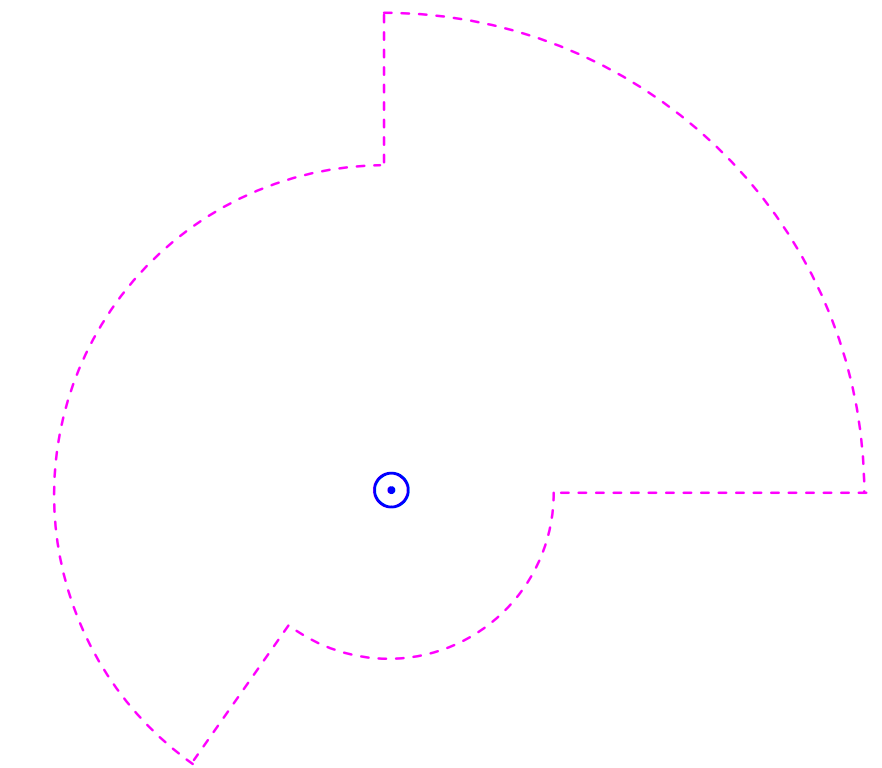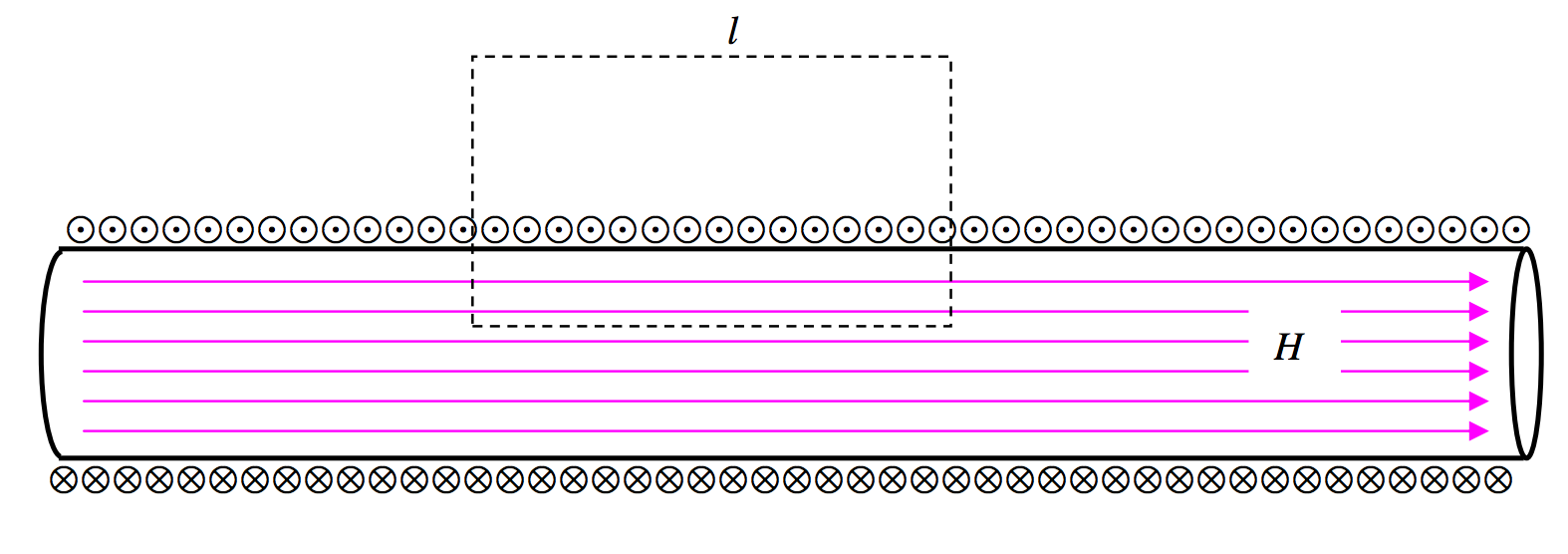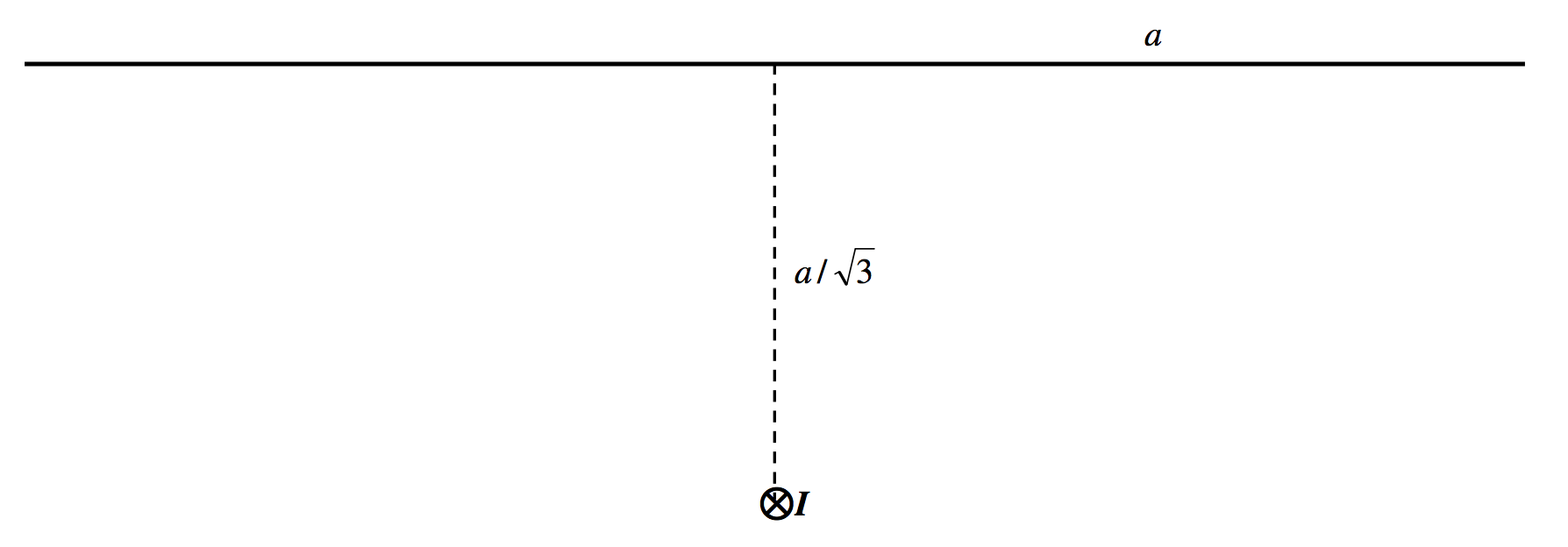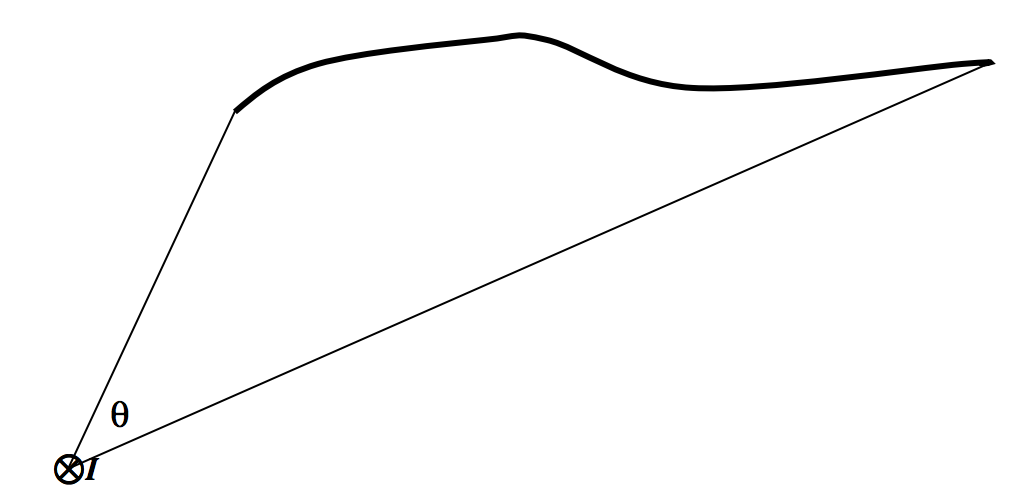$$\require{cancel}$$

# 6.11: Ampère’s Theorem

•• Contributed by Jeremy Tatum
• Emeritus Professor (Physics & Astronomy) at University of Victoria

In Section 1.9 we introduced Gauss’s theorem, which is that the total normal component of the $$D$$-flux through a closed surface is equal to the charge enclosed within that surface. Gauss’s theorem is a consequence of Coulomb’s law, in which the electric field from a point source falls off inversely as the square of the distance. We found that Gauss’s theorem was surprisingly useful in that it enabled us almost immediately to write down expressions for the electric field in the vicinity of various shapes of charged bodies without going through a whole lot of calculus.

Is there perhaps a similar theorem concerned with the magnetic field around a current-carrying conductor that will enable us to calculate the magnetic field in its vicinity without going through a lot of calculus? There is indeed, and it is called Ampère’s Theorem.$$\text{FIGURE VI.9}$$

In Figure $$\text{VI.9}$$ there is supposed to be a current $$I$$coming towards you in the middle of the circle. I have drawn one of the magnetic field lines – a dashed line of radius $$r$$. The strength of the field there is $$H = I/(2\pi r)$$. I have also drawn a small elemental length $$ds$$ on the circumference of the circle. The line integral of the field around the circle is just $$H$$ times the circumference of the circle. That is, the line integral of the field around the circle is just $$I$$. Note that this is independent of the radius of the circle. At greater distances from the current, the field falls off as $$1/r$$, but the circumference of the circle increases as $$r$$, so the product of the two (the line integral) is independent of $$r$$.$$\text{FIGURE VI.10}$$

Consequently, if I calculate the line integral around a circuit such as the one shown in Figure $$\text{VI.10}$$, it will still come to just $$I$$. Indeed it doesn’t matter what the shape of the path is. The line integral is $$\int \textbf{H}\cdot \textbf{ds}$$. The field $$\textbf{H}$$ at some point is perpendicular to the line joining the current to the point, and the vector $$\textbf{ds}$$ is directed along the path of integration, and $$\textbf{H}\cdot \textbf{ds}$$ is equal to $$H$$ times the component of $$\textbf{ds}$$ along the direction of $$\textbf{H}$$, so that, regardless of the length and shape of the path of integration:

The line integral of the field $$\textbf{H}$$ around any closed path is equal to the current enclosed by that path.

This is Ampère’s Theorem.

So now let’s do the infinite solenoid again. Let us calculate the line integral around the rectangular amperian path shown in Figure $$\text{VI.11}$$. There is no contribution to the line integral along the vertical sides of the rectangle because these sides are perpendicular to the field, and there is no contribution from the top side of the rectangle, since the field there is zero (if the solenoid is infinite). The only contribution to the line integral is along the bottom side of the rectangle, and the line integral there is just $$Hl$$, where $$l$$ is the length of the rectangle. If the number of turns of wire per unit length along the solenoid is $$n$$, there will be $$nl$$ turns enclosed by the rectangle, and hence the current enclosed by the rectangle is $$nlI$$, where $$I$$ is the current in the wire. Therefore by Ampère’s theorem, $$Hl = nlI$$, and so $$H = nI$$, which is what we deduced before rather more laboriously. Here $$H$$ is the strength of the field at the position of the lower side of the rectangle; but we can place the rectangle at any height, so we see that the field is $$nI$$ anywhere inside the solenoid. That is, the field inside an infinite solenoid is uniform.$$\text{FIGURE VI.11}$$

It is perhaps worth noting that Gauss’s theorem is a consequence of the inverse square diminution of the electric field with distance from a point charge, and Ampère’s theorem is a consequence of the inverse first power diminution of the magnetic field with distance from a line current.

Example $$\PageIndex{1}$$

Here is an example of the calculation of a line integral (Figure $$\text{VI.12}$$)An electric current $$I$$ flows into the plane of the paper at the origin of coordinates. Calculate the line integral of the magnetic field along the straight line joining the points $$(0 , a) \text{ and }(a , a)$$.

In Figure $$\text{VI.13}$$ I draw a (circular) line of force of the magnetic field $$\textbf{H}$$, and a vector $$\textbf{dx}$$ where the line of force crosses the straight line of interest.The line integral along the elemental length $$dx$$ is $$\textbf{H}\cdot \textbf{dx} = H\, dx\, \cos q$$ . Here $$H=\frac{I}{2\pi (a^2+x^2)^{1/2}}$$ and $$\cos \theta =\frac{a}{(a^2+x^2)^{1/2}}$$, and so the line integral along $$\textbf{dx}$$ is $$\frac{aI\,dx}{2\pi (a^2+x^2)}$$. Integrate this from $$x = 0\text{ to }x = a$$ and you will find that the answer is $$I/8$$.

Figure $$\text{VI.14}$$ shows another method. The line integral around the square is, by Ampère’s theorem, $$I$$, and so the line integral an eighth of the way round is $$I/8$$.

You will probably immediately feel that this second method is much the better and very “clever”. I do not deny this, but it is still worthwhile to study carefully the process of line integration in the first method.Example $$\PageIndex{2}$$

An electric current $$I$$ flows into the plane of the paper. Calculate the line integral of the magnetic field along a straight line of length $$2a$$ whose mid-point is at a distance $$a/\sqrt{3}$$ from the current.If you are not used to line integrals, I strongly urge you to do it by integration, as we did in the previous example. Some readers, however, will spot that the line is one side of an equilateral triangle, and so the line integral along the line is just $$\frac{1}{3}I$$.

We can play this game with other polygons, of course, but it turns out to be even easier than that.

For example:Show, by integration, that the line integral of the magnetic $$H$$-field along the thick line is just $$\frac{\theta}{2\pi}$$ times $$I$$.

After that it won’t take long to convince yourself that the line integral along the thick line in the drawing below is also $$\frac{\theta}{2\pi}$$ times $$I$$.Example $$\PageIndex{3}$$

A straight cylindrical metal rod (or a wire for that matter) of radius a carries a current $$I$$. At a distance $$r$$ from the axis, the magnetic field is clearly $$I/(2\pi r)\text{ if }r > a$$. But what is the magnetic field inside the rod at a distance r from the axis, $$r < a$$?$$\text{FIGURE VI.15}$$

Figure $$\text{VI.15}$$ shows the cross-section of the rod, and I have drawn an amperian circle of radius $$r$$. If the field at the circumference of the circle is $$H$$, the line integral around the circle is $$2\pi rH$$. The current enclosed within the circle is $$Ir^2/a^2$$. These two are equal, and therefore $$H = Ir/(2\pi a^2)$$.

# More and More Examples

In the above example, the current density was uniform. But now we can think of lots and lots of examples in which the current density is not uniform. For example, let us imagine that we have a long straight hollow cylindrical tube of radius $$a$$, perhaps a linear particle accelerator, and the current density J (amps per square metre) varies from the middle (axis) of the cylinder to its edge according to $$J(r)=J_0(1-r/a)$$. The total current is, of course, $$I=2\pi \int_0^a J(r)r\,dr=\frac{1}{3}\pi a^2 J_0$$ and the mean current density is $$\overline J = \frac{1}{3}J_0$$.

The question, however, is: what is the magnetic field $$H$$ at a distance $$r$$ from the axis? Further, show that the magnetic field at the edge (circumference) of the cylinder is $$\frac{1}{6}J_0 a$$, and that the field reaches a maximum value of $$\frac{3}{16}J_0 a$$ at $$r=\frac{3}{4}a$$.

Well, the current enclosed within a distance $$r$$ from the axis is

$\nonumber I=2\pi \int_0^r J(x)x\,dx = \pi J_0 r^2(1-\frac{2r}{3a}),$

and this is equal to the line integral of the magnetic field around a circle of radius $$r$$, which is $$2\pi rH$$. Thus

$\nonumber H=\frac{1}{2}J_0 r (1-\frac{2r}{3a}).$

At the circumference of the cylinder, this comes to $$\frac{1}{6}J_0 a$$. Calculus shows that $$H$$ reaches a maximum value of $$\frac{3}{16}J_0 a$$ at $$r=\frac{3}{4}a$$. The graph below shows $$H/(J_0a)$$ as a function of $$x/a$$.Having whetted our appetites, we can now try the same problem but with some other distributions of current density, such as

$\textbf{1}:J(r)=J_0\left ( 1-\frac{kr}{a}\right ); \textbf{ 2}:J(r)=J_0\left ( 1-\frac{kr^2}{a^2}\right ); \textbf{ 3}:J(r)=J_0\sqrt{1-\frac{kr}{a}}; \textbf{ 4}:J(r)=J_0\sqrt{1-\frac{kr^2}{a^2}}.\nonumber$

The mean current density is $$\overline J =\frac{2}{a^2}\int_0^a rJ(r)\,dr$$, and the total current is $$\pi a^2$$ times this.

The magnetic field is $$H(r)=\frac{1}{r}\int_0^r xJ(x)\,dx$$.

Here are the results:

1. $J=J_0 \left ( 1-\frac{kr}{a}\right ),\quad \overline J = J_0 \left ( 1-\frac{2k}{3}\right ),\quad H(r)=J_0\left ( \frac{r}{2}-\frac{kr^2}{3a}\right ).$$$H$$ reaches a maximum value of $$\frac{3J_0 a}{16k}\text{ at }r=\frac{3a}{4k}$$, but this maximum occurs inside the cylinder only if $$k>\frac{3}{4}$$.

2. $J=J_0\left ( 1-\frac{kr^2}{a^2}\right ),\quad \overline J = J_0(1-\frac{1}{2}k),\quad H(r)=J_0\left ( \frac{r}{2}-\frac{kr^3}{4a^2}\right ).$$$H$$ reaches a maximum value of $$\sqrt{\frac{2}{27k}}J_0\text{ at }\frac{r}{a}=\sqrt{\frac{2}{3k}}$$, but this maximum occurs inside the cylinder only if $$k>\frac{2}{3}$$.

3. $J=J_0\sqrt{1-\frac{kr}{a}},\quad \overline J = \frac{J_0}{15k^2}[8-20(1-k)^{3/2}+12(1-k)^{5/2}],\quad H(r)=\frac{2J_0 a^2}{15k^2r}\left [ 2-5 \left ( 1-\frac{kr}{a}\right )^{3/2}+3\left ( 1-\frac{kr}{a}\right )^{5/2}\right ] .$I have not calculated explicit formulas for the positions and values of the maxima. A maximum occurs inside the cylinder if $$k > 0.908901$$.

4. $J=J_0\sqrt{1-\frac{kr^2}{a^2}},\quad \overline J = \frac{2J_0}{3k}\left [ 1-(1-k)^{3/2} \right ],\quad H(r)=\frac{J_0 a^2}{3kr}\left [ 1-\left ( 1-\frac{kr^2}{a^2}\right )^{3/2} \right ] .$A maximum occurs inside the cylinder if $$k > 0.866025$$.

In all of these cases, the condition that there shall be no maximum $$H$$ inside the cylinder – that is, between $$r = 0\text{ and }r = a$$ - is that $$\frac{J(a)}{\overline J}>\frac{1}{2}$$. I believe this to be true for any axially symmetric current density distribution, though I have not proved it. I expect that a fairly simple proof could be found by someone interested.

$J=\frac{J_0}{1+x/a}\qquad J=\frac{J_0}{1+x^2/a^2}\qquad J=\frac{J_0}{\sqrt{1+x/a}}\nonumber$
$J=\frac{J_0}{\sqrt{1+x^2/a^2}} \qquad J=J_0e^{-x/a} \qquad J=J_0 e^{-x^2/a^2} \nonumber$